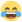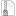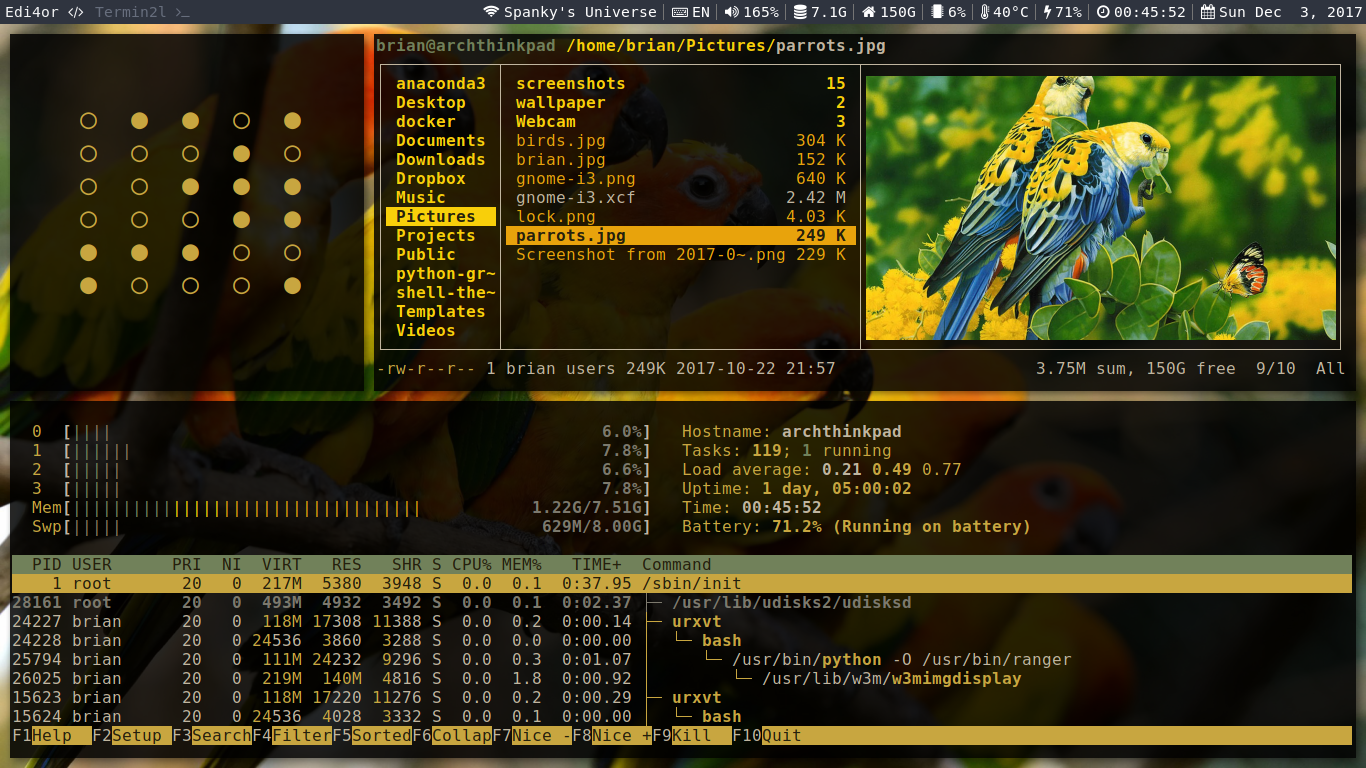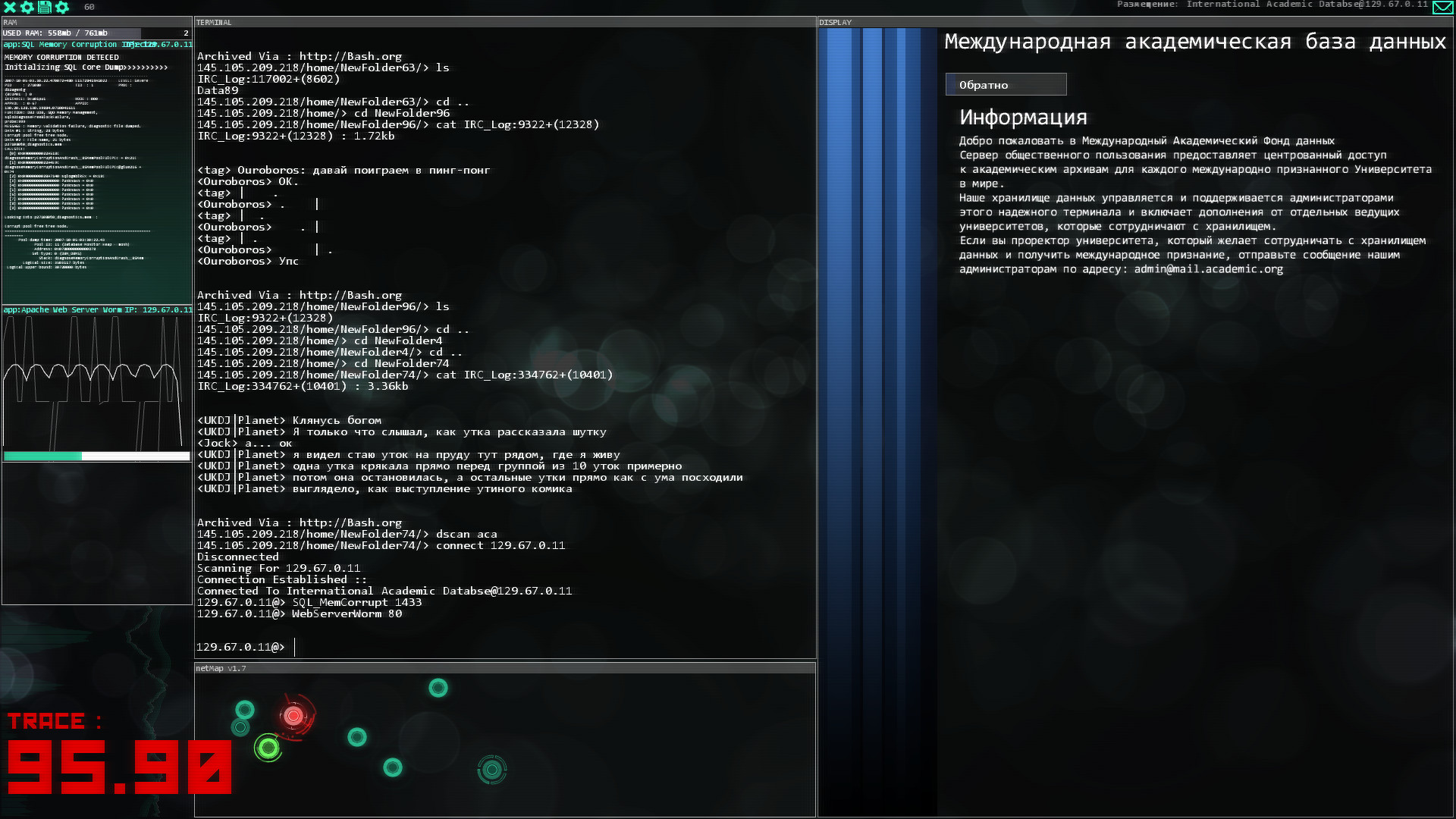• 0 Vote(s) - 0 Average
• 1
• 2
• 3
• 4
• 5

# Recursive Sequence of Death [Python]

We've been working on recursive sequences in python in classes lately, and I was a little surprised at how much math actually makes a difference in your ability to optimize code. I wrote a little demonstration for one of my assignments.

The fibonacci sequence makes no sense to me, but essentially, it goes like this.

0, 1, 1, 2, 3, 5, 8, 13, 21, ...

Each digit is the sum of the two previous digits. Pretty simple. We could definitely extrapolate this as far as we wanted to and get an infinitely large number of digits, but I won't go there. Apparently this is some sort of important sequence in computer science and I don't know why, but you should be able to see the pattern easily enough.

Say we wanted to know what, say, the 20th digit in the sequence was? It turns out that the method for finding this is a little bit more complicated than you'd think. It's rather tedious to go and actually add up all of the numbers. And particularly if you're going to use a recursive sequence, as fast as we think computers are these days, trying to go past about the 40th term or so in the sequence will crash my macbook because literally going through and generating this sequence is that tedious for a computer. Maybe yours might get a tad bit higher but I'd be willing to bet you won't get past 50 in Python.

Okay, but why in the world is this so freaking slow?

It's because it's recursive. If it wants to find the 5th number in the sequence, it first must find the 4th and the 3rd, and add those up. But because it doesn't know what the 4th and the 3rd are, in order to find the 4th, it must find the 3rd and the 2nd. And in order to find the 3rd, it must find the 2nd and the first. And it has to go through all of these steps before it can give a simple answer. Although this is easy for small numbers, as you can probably imagine, this starts to grow exponentially once you start finding, say, the 20th, or the 30th, or the 35th number in a sequence. The next thing you know, your computer can't even find the 40th term in a simple sequence without crashing, where you could probably do it by hand in a matter of a few minutes.

There are two ways to speed this up. Instead of calling the same function over and over again and creating a gigantic tree of function calls to recalculate the same data over and over and over again, you can either save what each digit is as you go, and only calculate if you haven't saved that particular digit yet, or you can use something called an exact solution (a simple formula you make out of the recursive sequence using some sort of voodoo magic) to do the trick. By saving these numbers, it prevents the results from growing exponentially because it never has to recalculate values it's gone over before. You can easily calculate, say, the 4000th term with this method.

I demonstrated all three methods with the following code. It calculates the 35th number in the sequence. Enough to take a few seconds on the recursive calculation, but not enough to crash my Macbook. Hopefully it won't crash yours.``````import sys

sys.setrecursionlimit(2000000000)

print ("Will evaluate three methods of calculating the fibonacci sequence at 35.\n")

#define a simple recursive fibonacci sequence.
def recursive_f(n):
"""Slow recursive function. n = the number to evaluate. """

if n == 0:
return 0     #base case
elif n == 1:
return 1     #another base case
else:
return recursive_f(n-1)+recursive_f(n-2)

#same as the previous function, but store previous values.
#this keeps the function from re-evaluating the same values.
def mem_f(n, mem=dict()):
"""Memoization recursive method. n = num to eval. Don't pass mem. """

if (n in mem):
return mem[n]   #welp we already saved a value. Don't re-evaluate.

if n == 0:
return 0    #base case
elif n == 1:
return 1    #base case
else:
value = mem_f(n-1, mem)+ mem_f(n-2, mem)
mem[n] = value  #store value
return value

#This solution is a little different. This is a formula that is not recursive.
#In theory python shouldn't crash, but large numbers are beyond python's range.
def fib_exact(n):
"""Calculated exact solution from second order sequence. n = num to eval."""

value1 = ((1 + (5**(0.5)))**n)
value2 = ((1 - (5**(0.5)))**n)
value3 = (2**n) * (5**(0.5))

result = (value1 - value2) // value3
return int(result)

#recursive with slow method. will take forever.
print ("Slow recursive method: ", end="")
print (str(recursive_f(35)) + "\n")

#recursive with fast method. Much faster.
print ("Fast recursive method: ", end="")
print(str(mem_f(35)) + "\n")

#Exact solution. The fastest of them all.
print ("Exact non-recursive method: ", end="")
print (str(fib_exact(35)))
``````

This will run in Python 3 if you want to save it into a .py file and run it. And I attached a file with this post too.

This page (yes that's a link) pretty much describes what we spent an hour or two doing in class to optimize this. It's my very first semester in this new program, I haven't even taken Calculus yet, and I already feel like my head is hurting.Needless to say, you can teach yourself to program, but it's hard to teach yourself everything. I feel that, even solving simple problems, I have learned a lot.Attached Filesfib2.py.zip (Size: 1.29 KB / Downloads: 140)
Saturn-Moon.com - Our next project...
haha nice! python is currently my favorite language just because of it's flexibility.

have fun learning to tame the snake"I reject your reality and subsitute my own." - Adam Savage, MythbustersHaha thank you! Yeah Python is pretty easy all things considered. It's so nice to code that I almost wish it was more popular in the web sphere of things. As much as I don't particularly care for PHP, it's what we use here because it's what is widely supported.

Java is probably going to be what I have to tackle next, unfortunately. And Java is no fun, from what little I have done so far.
Saturn-Moon.com - Our next project...
@Darth: ever try combining python with I3 and some Texmode User interface stuff?

if you've ever played hacknet it looks really close to the game's interface, crazy stuff"I reject your reality and subsitute my own." - Adam Savage, MythbustersHmm, that's the first I've really heard of it. I've done a fair amount of stuff in Turtle (trivially easy), might have to check it out.
Saturn-Moon.com - Our next project...
Using python programs while running this window manager.Compare to a screenshot of this game.crazy right?

but super hard core functional.
"I reject your reality and subsitute my own." - Adam Savage, MythbustersRecursion, as cool of a concept as it is, is honestly pretty useless for fib unless you're only going after small terms. So yeah, 5-25. Anything more risks a complete stack overflow.

Here's a recursive example of what the call stack will look like:
``````call fib(20)
call fib(19)
call fib(18)
call fib(17)
...
return 0, 1
}
}
}
}``````
Returning whatever value.

Now, iteratively:
``````uint_32 a, 0;
uint_32 b, 1;
uint_32 c;
uint_32 fib(int x){
for(int i = 0; i < x; i++){
c = a+b
a = b;
b = c;
}
return c;
}
fib(20)``````
There are probably errors in this, it's just pseudocode so scoping is probably f*** and I probably don't need to use 32bit integers for small terms.

But the call-stack will look more like:
``````call fib(20)
loop
}``````

There's more intensive computation in the one function, but there's no need for a massive call-stack, which in turn makes it run quite a bit quicker.
Recursion is a pretty solution, but that's about it.
Sounds interesting. Thanks for all of this information.Possibly Related Threads… Thread Author Replies Views Last Post Cython - Python at the Speed of C Darth-Apple 0 303 April 11th, 2020 at 1:07 PM Last Post: Darth-Apple Python programmers? Darth-Apple 8 4,530 March 8th, 2020 at 7:38 AM Last Post: SpookyZalost

Users browsing this thread: 1 Guest(s)

Makestation Theme/Design Selector